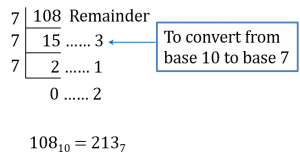# SPM Mathematics Trial 2021 (Kelantan), Paper 2 (Question 3 & 4)

Question 3 (4 marks):
Zulaikha is 1326 years old. Zulaikha’s age is twice the age of her daughter, Zahira. The ratio of Zahira’s age to the age of her younger brother, Ahmad is 7:6. Calculate the total age Zulaikha and her children in base 7.

Solution:
Zulaikha’s age,
1326 = (1 × 62) + (3 × 61) + (2 × 60)
= 36 + 18 + 2
= 56 years old

Zahira’s age = 56 ÷ 2
= 28 years old

Zahira : Ahmad = 7 : 6
= (7 × 4) : (6 × 4)
= 28 : 24
Thus, Ahmad = 24 years old

Total age = 56 + 28 + 24
= 108 years oldThus, the total age of Zulaikha and her children in base 7 = 2137

Question 4 (4 marks):
In a furniture exhibition, there is a piece of round-shaped window decorated with a small circle carving the same size as Diagram 2. The radius of the window is 72 cm. Calculate the area of the uncarved area. (Use π=22/7 )Diagram 2

Solution:
Radius of small circle = 72 ÷ 3 = 24 cm